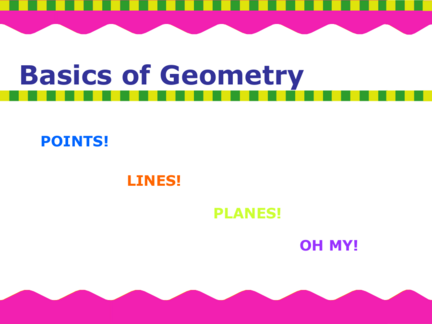# Basics of Geometry ( POINTS, LINES, PLANES! )Contributed by:1. What are the undefined terms in geometry?
2. What concepts present the foundations of geometry?
3. Can you sketch the intersection of lines and planes?
1. Basics of Geometry
Basics of Geometry
POINTS!
LINES!
PLANES!
OH MY!
2. Basics of Geometry
• What are the undefined terms in geometry?
• What concepts present the foundations of
geometry?
• Can you sketch the intersection of lines and
planes?
These questions (and much more!!) will be
answered by the end of this presentation.
3. Basics of Geometry
Undefined Terms?
The terms points, lines, and planes are the foundations of
geometry, but…
point, line, and plane are all what we call undefined terms.
How can that be?
Well, any definition we could give them would depend on the
definition of some other mathematical idea that these three
terms help define. In other words, the definition would be
4. Basics of Geometry
Point
• Has no dimension
• Usually represented by a small dot
A
The above is called point A. Note the point
is represented with a capital letter.
5. Basics of Geometry
Line
• Extend in one dimension.
• Represented with straight line with two arrowheads to
indicate that the line extends without end in two directions.
l This is Line l, (using the lower case
script letter) or symbolically we call it AB
A
B NOTICE: The arrowheads are in
both directions on the symbol AB
6. Basics of Geometry
Plane
• Extend in two dimensions.
• Represented by a slanted 4 sided figure, but you must
envision it extends without end, even though the
representation has edges.
A M This is Plane M or plane ABC (be
C sure to only use three of the
B points when naming a plane)
7. Basics of Geometry
Undefined Concepts
• Collinear points are points that lie on the
same line.
l
A
B Points A, B and C are collinear.
C
8. Basics of Geometry
Undefined Concepts
• Coplanar points are points that lie on the
same plane.
A
C Points A, B and C are coplanar.
B
9. Basics of Geometry
Line Segment
Let’s look at the idea of a point in between two other points on a line.
Here is line AB, or recall A B
symbolically AB
The line segment does not
extend without end. It has
endpoints, in this case A and
B. The segment contains all
the points on the line
between A and B
This is segment AB
Notice the difference in
the symbolic notation!
10. Basics of Geometry
Let’s look at a ray:
A is called the initial
point The initial point is
always the first
A B letter in naming a
ray. Notice the
difference in
Ray AB extends in symbols from both
one direction a line and segment.
without end.
Symbolized by AB
11. Basics of Geometry
Not all symbols are created equal!
AB is the same as BA A B
AB is the same as BA A B
BUT…
12. Basics of Geometry
The ray is different! Initial point 1st
AB is not the same as BA
A B AB
A B BA
Notice that the initial point is listed first in the symbol. Also
note that the symbolic ray always has the arrowhead on the right
regardless of the direction of the ray.
13. Basics of Geometry
Opposite Rays
If C is between A and B,
A C B
then CA and CB are opposite rays.
C is the common initial point for the rays!
14. Basics of Geometry
Angles
Rays are important because they help us define something very
important in geometry…Angles!
An angle consists of two different rays that have the same initial
point. The rays are sides of the angles. The initial point is called the
vertex. Notation: We denote an angle with
three points and  symbol. The
vertex B middle point is always the vertex.
We can also name the angle with
sides just the vertex point. This angle can
A be denoted as:
C BAC , CAB, or A
15. Basics of Geometry
Classifying Angles
Angles are classified as acute, right, obtuse, and straight,
according to their measures. Angles have measures greater
than 0° and less or equal to 180°.
A A A A
Acute angle Right angle Obtuse angle Straight angle
0°< m  A < 90° m  A = 90° 90°< m  A < 180° m  A = 180°
16. Basics of Geometry
Intersections of lines and planes
• Two or more geometric figures intersect if they have
one or more points in common.
• The intersection of the figures is the set of points the
figure has in common
Think How do 2 line intersect?
!! How do 2 planes intersect?
What about a line and a plane?
17. Basics of Geometry
Modeling Intersections
To think about the questions on the last slide lets look at the following…
Point E is
Two lines E the
intersect at a intersection
point, like here of plane H
A F and line EC
at point A. B
D
H C
G
Line BF is the intersection of the
planes G and H.
18. Basics of Geometry